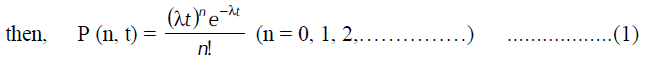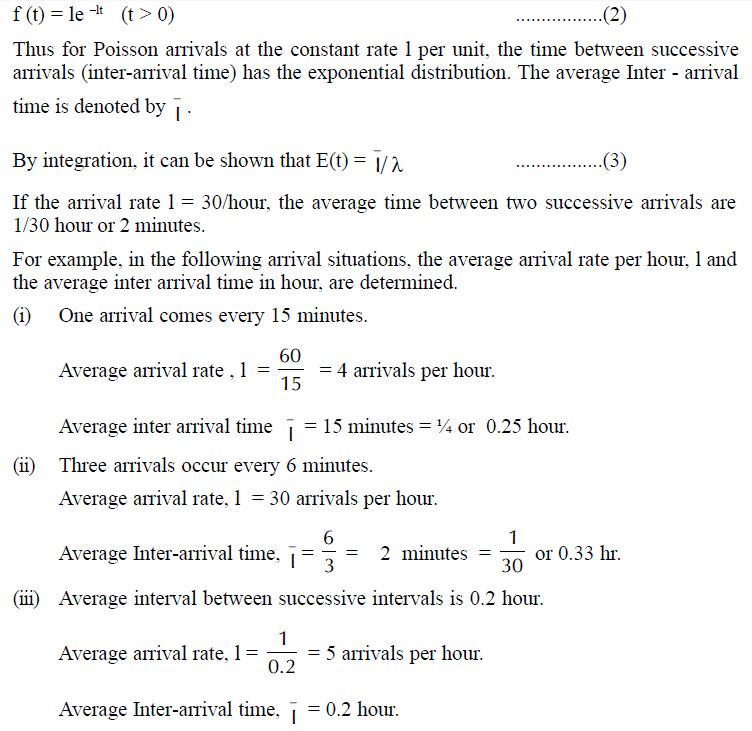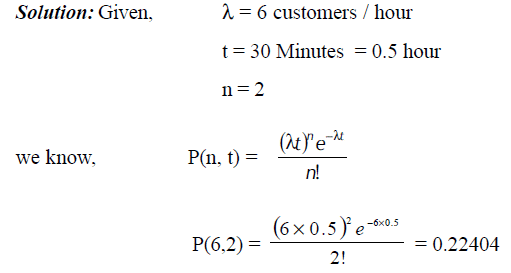# Application of Poisson and Exponential distribution in estimating Arrival Rate and Service Rate

Both the Poisson and Exponential distributions play a prominent role in queuing theory. The Poisson distribution counts the number of discrete events in a fixed time period; it is closely connected to the exponential distribution, which (among other applications) measures the time between arrivals of the events. The Poisson distribution is a discrete distribution; the random variable can only take nonnegative integer values. The exponential distribution can take any (nonnegative) real value.

Considering a problem of determining the probability of arrivals being observed during a time interval of length t, where the following assumptions are made.

1. Probability that an arrival is observed during a small time interval (say of length v) is proportional to the length of interval. Let the proportionality constant be l, so that the probability is lv.
2. Probability of two or more arrivals in such a small interval is zero.
3. Number of arrivals in any time interval is independent of the number in nonoverlapping time interval.

These assumptions may be combined to yield what probability distributions are likely to be, under Poisson distribution with exactly n customers in the system.

Suppose function P is defined as follows:

P (n customers during period t) = the probability that n arrivals will be observed in a time interval of length tThis is the Poisson probability distribution for the discrete random variable n, the number of arrivals, where the length of time interval, t is assumed to be given. This situation in queuing theory is called Poisson arrivals. Since the arrivals alone are considered (not departures), it is called a pure birth process.

The time between successive arrivals is called inter-arrival time. In the case where the number of arrivals in a given time interval has Poisson distribution, inter-arrival times can be shown to have the exponential distribution. If the inter-arrival times are independent random variables, they must follow an exponential distribution with density f(t) where,### Poisson and Exponential Distribution Practice Problems

The practice problems of poisson and exponential distributions are given below

Example: In a factory, the machines break down and require service according to a Poisson distribution at the average of four per day. What is the probability that exactly six machines break down in two days?In the output screen, for n = 6 the probability, Pn is given as 0.12214.

Example: On an average, 6 customers arrive in a coffee shop per hour. Determine the probability that exactly 3 customers will reach in a 30 minute period, assuming that the arrivals follow Poisson distribution.Similarly, when the time taken to serve different customers are independent, the probability that no more than t periods would be required to serve a customer is given by exponential distribution as follows:

p(not more than t time period) = 1 – e– mt where m = average service rate

Example: A manager of a fast food restaurant observes that, an average of 9 customers is served by a waiter in a one-hour time period. Assuming that the service time has an exponential distribution, what is the probability that

1. A customer shall be free within 12 minutes.
2. A customer shall be serviced in more than 25 minutes.

Solution:

(a) Given, μ = 9 customers / hour
t = 15 minutes = 0.25 hour
Therefore, p (less than 15 minutes) = l – e– mt
= 1– e– 9 × 0.25
= 0.8946
(b) Given, μ = 9 customers / hour
t = 25 minutes = 0.4166 hour
Therefore, P (more than 25 minutes) = l – e– mt
= 1– e– 9 × 0.4166
= 0.0235

To analyze queuing situations, the questions of interest that are typically concerned with measures of queuing system performance include,

• What will be the waiting time for a customer before service is complete?
• What will be the average length of the queue?
• What will be the probability that the queue length exceeds a certain length?
• How can a system be designed at minimum total cost?
• How many servers should be employed?
• Should priorities of the customers be considered?
• Is there sufficient waiting area for the customers?

## One thought on “Application of Poisson and Exponential distribution in estimating Arrival Rate and Service Rate”

error: Content is protected !!